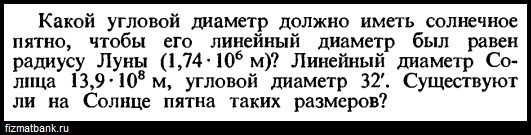# Как решить вопрос, связанный с телесным углом и т. д.?How do we attempt such questions? One more question which I have:-

1st question:
because the angles are small, the distance of the sun is L_S = 13,9\cdot 10^8/32’=1.493\cdot 10^{11}\space\text{m}. I have preliminarily translated this angle in radians. The desired angular diameter is \theta_\text{sunspot} = 1.74\cdot10^6/L_S=1.165\cdot10^{-5}\space\text{rad}=2.4’’

2nd question:
for any problem involving solid angles it is necessary to know the formula

d\Omega = \iint_S\sin\varphi d\theta d\varphi,

when the coordinates of an element dS are described in spherical coordinates

4 лайка

Oh, I see… I need to know spherical coordinates. Thank you!